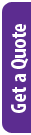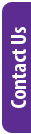## Details

Statistics and data fuel business with information and insights and programming has made working with these stats easier and more powerful. R is a programming language developed for statistical computing to make data analysis accessible and effective.

In this course we will use the R programming language to:

• Work with probability
• Understand factors in probability
• Calculate combinations and permutations
• Work with continuous variables
• Work with random variables
• Apply central limit theorem
• Apply statistics to real-world scenarios

### Delivery Methods

Leading Training is focusing on providing virtual training courses for the foreseeable future and will only consider in-person and classroom training on request, with a required minimum group size of four delegates. We remain committed to offering training that is fast, focused and effective.

Delivery Method Duration Price (excl. VAT)
Fulltime 4 Days R 11,596.00
Webinar 4 Days R 9,400.00

### Discounts Available

Save up to 10% by booking and paying 10 business days before the course.

### Brochure:

Information may change without notice.

## Audience

• Data scientists
• Programmers
• Engineers
• Scientists

## Pre-Requisites

Leading Training's Introduction to R or equivalent knowledge

## Course Outline / Curriculum

Introduction to statistics with R

Probability

• Discrete probability
• Relative frequency
• Notation
• Probability distributions
• Monte Carlo simulations for categorical data
• Setting the random seed
• With and without replacement
• Independence
• Conditional probabilities
• Multiplication rule
• Multiplication rule under independence
• Combinations and permutations
• Monte Carlo example
• Examples
• Monty Hall problem
• Birthday problem
• Infinity in practice
• Exercises
• Continuous probability
• Theoretical continuous distributions
• Theoretical distributions as approximations
• The probability density
• Monte Carlo simulations for continuous variables
• Continuous distributions
• Exercises

Random variables

• Random variables
• Sampling models
• The probability distribution of a random variable
• Distributions versus probability distributions
• Notation for random variables
• The expected value and standard error
• Population SD versus the sample SD
• Central Limit Theorem
• How large is large in the Central Limit Theorem?
• Statistical properties of averages
• Law of large numbers
• Misinterpreting law of averages
• Exercises
• Case study: The Big Short
• Interest rates explained with chance model
• The Big Short
• Exercises

## Schedule Dates and Booking

There are currently no scheduled dates.

Please note that this course needs a minimum of 6 delegates to schedule a course. You can choose to be added to the waiting list by clicking the button below and we will contact you when we have enough delegates interested. Should we not get enough delegates, we will refund or credit your paid booking.

Should you need this course urgently, the following options are available:

1. Pay for 6 delegates (whether you have them or not) and we will schedule the course as soon as possible.
2. If you have fewer delegates and cannot pay for 6, we can negotiate a shortened course where some of the time will be spent in blended learning - watching videos and doing tutorials and exercises with some contact time with the trainer. We would want to discuss what your core needs are so that we cover those aspects. You need to have paid for 3 delegates at least.